# Intermediate Algebra Tutorial 37

Intermediate Algebra

WTAMU > Virtual Math Lab > Intermediate AlgebraLearning Objectives

After completing this tutorial, you should be able to:
1. Find the principal nth root of an expression.
2. Find the nth root of an expression raised to the nth power.Introduction

In this tutorial we will be looking at radicals (or roots).  Basically, the root of an expression is the reverse of raising it to a power.  For example, if you want the square root of an expression, then you want another expression, such that, when you square it, you get what is inside the square root.  This concept carries through to all roots.Tutorial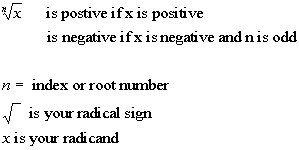When looking for the nth radical or nth root, you want the expression that, when you raise it to the nth power, you would get the radicand (what is inside the radical sign).

When there is no index number, n, it is understood to be a 2 or square root.  For example: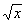= principal square root of x.

Note that NOT EVERY RADICAL is a square root.  If there is an index number n other than the number 2, then you have a root other than a square root.Example 1: Simplify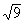.

The thought behind this is that we are looking for the square root of 9. This means that, we are looking for a number that when we square it, we get 9.

What do you think it is?

Let's find out if you are right: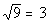Since 3 squared is 9, 3 is the square root of 9.

Note that we are only interested in the principal root and since 9 is positive and there is not a sign in front of the radical, our answer is positive 3.  If there had been a negative in front of the radical, our answer would have been -3.Example 2: Simplify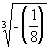.

Now we are looking for the third or cube root of -1/8. This means we are looking for a number that, when we cube it, we get -1/8.

What do you think it is?

Let's find out if you are right: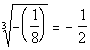Since -1/2 cubed is -1/8, our answer is going to be -1/2.Example 3: Simplify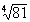.

Now we are looking for the fourth root of 81. This means that we are looking for a number that, when we raise it to the fourth power,  we get 81.

What do you think it is?

Let's find out if you are right: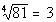Since 3 raised to the fourth power is 81, our answer is going to be 3.Example 4: Simplify.  Assume that variables represent positive real numbers.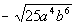The thought behind this is that we are looking for the square root of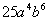. This means that we are looking for an expression that, when we square it, we getWhat do you think it is?

Let's find out if you are right: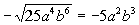Since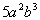squared isand we have a negative in front of the square root, -is the negative square root ofExample 5: Simplify.  Assume that variables represent positive real numbers.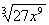The thought behind this is that we are looking for the cube root of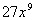. This means that we are looking for an expression that, when we cube it, we get.

What do you think it is?

Let's find out if you are right: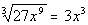Since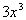cubed isis the cube root of.Example 6: Simplify.  Assume that variables represent positive real numbers.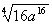The thought behind this is that we are looking for the fourth root of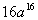. This means that we are looking for an expression that, when we raise it to the fourth power, it we getWhat do you think it is?

Let's find out if you are right: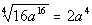Since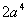raised to the fourth power isis the fourth root of.Example 7: Simplify.  Assume that variables represent positive real numbers.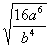The thought behind this is that we are looking for the square root of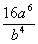. This means that we are looking for an expression that, when we square it,  we getWhat do you think it is?

Let's find out if you are right: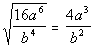Since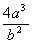squared isis the square root of.Example 8: Simplify.  Assume that variables represent positive real numbers.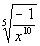The thought behind this is that we are looking for the fifth root of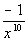. This means that we are looking for an expression that, when we raise it to the fifth power, we getWhat do you think it is?

Let's find out if you are right: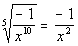Since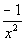raised to the fifth power  isis the fifth root of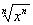rule

If n is an even positive integer, then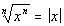If n is an odd positive integer, then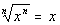The above examples all said that the variables were positive real numbers.  But if a problem does not indicate that, then you need to assume that we are dealing with both positive and negative real numbers and use this rule.Example 9: Simplify.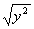Since it didn't say that y is positive, we have to assume that it can be either positive or negative.  And since the root number and exponent are equal, then we can use therule.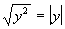Since the root number and the exponent inside are equal and are the even number 2, then we need to put an absolute value around y for our answer.

The reason for the absolute value is that we do not know if y is positive or negative.  So if we put y as our answer and it was negative, it would not be a true statement.

For example if y was -5, then -5 squared would be 25 and the square root of 25 is 5, which is not the same as -5.  The only time that you do not need the absolute value on a problem like this is if it stated that the variable is positive as it did on examples 1 - 8 above.Example 10: Simplify.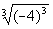Since the root number and exponent are equal, then we can use therule.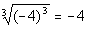This time our root number and exponent were both the odd number 3.  When an odd numbered root and exponent match, then the answer is the base whether it is negative or positive.Example 11: Simplify.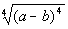Since it didn't say that a or b are positive, we have to assume that they can be either positive or negative.  Since the root number and exponent are equal, then we can use therule.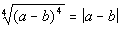Since the root number and the exponent inside are equal and are the even number 4, then we need to put an absolute value around a - b for our answer.  The reason for the absolute value is that we do not know if a or b are positive or negative.  So if we put a - b as our answer and it was negative, it would not be a true statement.Practice Problems

These are practice problems to help bring you to the next level.  It will allow you to check and see if you have an understanding of these types of problems. Math works just like anything else, if you want to get good at it, then you need to practice it.  Even the best athletes and musicians had help along the way and lots of practice, practice, practice, to get good at their sport or instrument.  In fact there is no such thing as too much practice.

To get the most out of these, you should work the problem out on your own and then check your answer by clicking on the link for the answer/discussion for that  problem.  At the link you will find the answer as well as any steps that went into finding that answer.Practice Problems 1a - 1d: Simplify.  Assume that variables represent positive real numbers.

1a.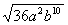1b.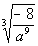1c.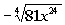1d.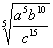Practice Problems 2a - 2b: Simplify.

2a.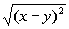2b.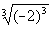Need Extra Help on these Topics?

Go to Get Help Outside the Classroom found in Tutorial 1: How to Succeed in a Math Class for some more suggestions.Last revised on July 19, 2011 by Kim Seward.